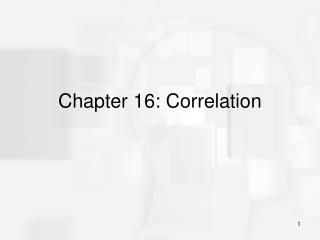DownloadDownload PresentationChapter 16: Correlation

# Chapter 16: Correlation

Télécharger la présentation## Chapter 16: Correlation

- - - - - - - - - - - - - - - - - - - - - - - - - - - E N D - - - - - - - - - - - - - - - - - - - - - - - - - - -
##### Presentation Transcript

1. Chapter 16: Correlation

2. Correlations: Measuring and Describing Relationships • A correlation is a statistical method used to measure and describe the relationship between two variables. • A relationship exists when changes in one variable tend to be accompanied by consistent and predictable changes in the other variable.

3. Correlations: Measuring and Describing Relationships (cont.) • A correlation typically evaluates three aspects of the relationship: • the direction • the form • the degree

4. Correlations: Measuring and Describing Relationships (cont.) • The direction of the relationship is measured by the sign of the correlation (+ or -). A positive correlation means that the two variables tend to change in the same direction; as one increases, the other also tends to increase. A negative correlation means that the two variables tend to change in opposite directions; as one increases, the other tends to decrease.

5. Correlations: Measuring and Describing Relationships (cont.) • The most common form of relationship is a straight line or linear relationship which is measured by the Pearson correlation.

6. Correlations: Measuring and Describing Relationships (cont.) • The degree of relationship (the strength or consistency of the relationship) is measured by the numerical value of the correlation. A value of 1.00 indicates a perfect relationship and a value of zero indicates no relationship.

7. Correlations: Measuring and Describing Relationships (cont.) • To compute a correlation you need two scores, X and Y, for each individual in the sample. • The Pearson correlation requires that the scores be numerical values from an interval or ratio scale of measurement. • Other correlational methods exist for other scales of measurement.

8. The Pearson Correlation • The Pearson correlation measures the direction and degree of linear (straight line) relationship between two variables. • To compute the Pearson correlation, you first measure the variability of X and Y scores separately by computing SS for the scores of each variable (SSX and SSY). • Then, the covariability (tendency for X and Y to vary together) is measured by the sum of products (SP). • The Pearson correlation is found by computing the ratio, SP/(SSX)(SSY) .

9. The Pearson Correlation (cont.) • Thus the Pearson correlation is comparing the amount of covariability (variation from the relationship between X and Y) to the amount X and Y vary separately. • The magnitude of the Pearson correlation ranges from 0 (indicating no linear relationship between X and Y) to 1.00 (indicating a perfect straight-line relationship between X and Y). • The correlation can be either positive or negative depending on the direction of the relationship.

10. The Spearman Correlation • The Spearman correlation is used in two general situations: (1) It measures the relationship between two ordinal variables; that is, X and Y both consist of ranks. (2) It measures the consistency of direction of the relationship between two variables. In this case, the two variables must be converted to ranks before the Spearman correlation is computed.

11. The Spearman Correlation (cont.) The calculation of the Spearman correlation requires: 1. Two variables are observed for each individual. 2. The observations for each variable are rank ordered. Note that the X values and the Y values are ranked separately. 3. After the variables have been ranked, the Spearman correlation is computed by either: a. Using the Pearson formula with the ranked data. b. Using the special Spearman formula (assuming there are few, if any, tied ranks).

12. The Point-Biserial Correlation and the Phi Coefficient • The Pearson correlation formula can also be used to measure the relationship between two variables when one or both of the variables is dichotomous. • A dichotomous variable is one for which there are exactly two categories: for example, men/women or succeed/fail.

13. The Point-Biserial Correlation and the Phi Coefficient (cont.) With either one or two dichotomous variables the calculation of the correlation precedes as follows: 1. Assign numerical values to the two categories of the dichotomous variable(s). Traditionally, one category is assigned a value of 0 and the other is assigned a value of 1. 2. Use the regular Pearson correlation formula to calculate the correlation.

14. The Point-Biserial Correlation and the Phi Coefficient (cont.) • In situations where one variable is dichotomous and the other consists of regular numerical scores (interval or ratio scale), the resulting correlation is called a point-biserial correlation. • When both variables are dichotomous, the resulting correlation is called a phi-coefficient.

15. The Point-Biserial Correlation and the Phi Coefficient (cont.) • The point-biserial correlation is closely related to the independent-measures t test introduced in Chapter 10. • When the data consists of one dichotomous variable and one numerical variable, the dichotomous variable can also be used to separate the individuals into two groups. • Then, it is possible to compute a sample mean for the numerical scores in each group.

16. The Point-Biserial Correlation and the Phi Coefficient (cont.) • In this case, the independent-measures t test can be used to evaluate the mean difference between groups. • If the effect size for the mean difference is measured by computing r2 (the percentage of variance explained), the value of r2 will be equal to the value obtained by squaring the point-biserial correlation.